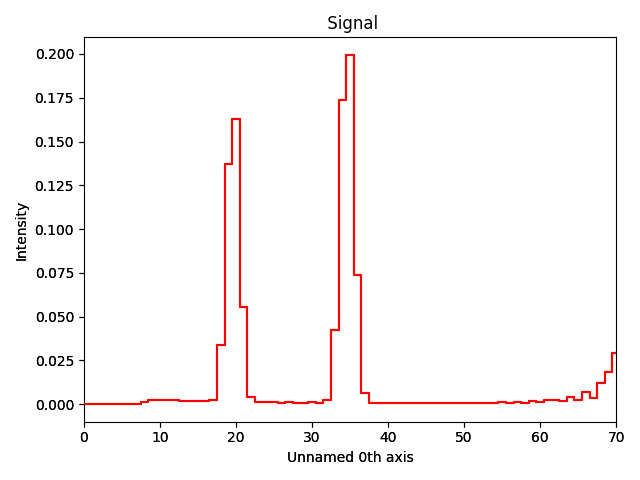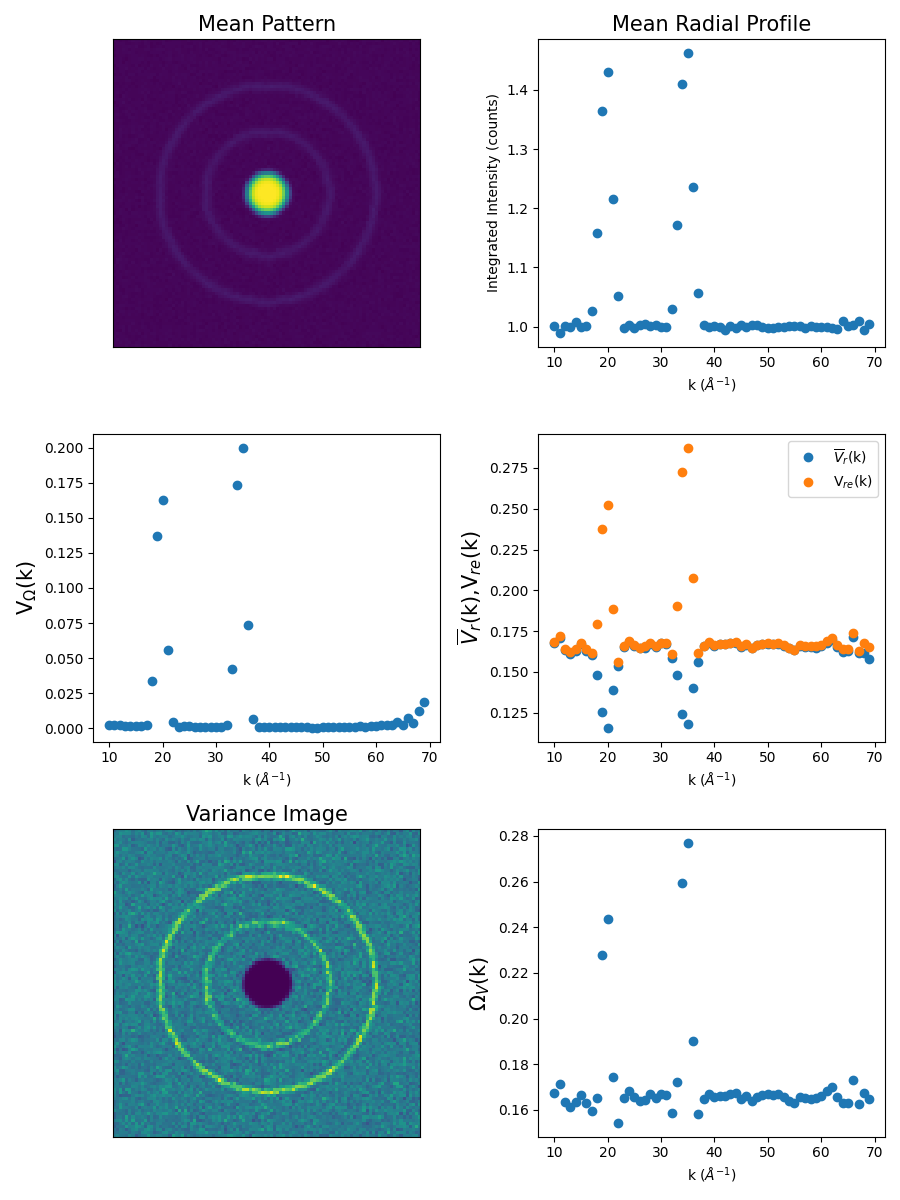# Fluctuation electron microscopy analysis¶

`fem_analysis()`

Pixelated STEM can be used as a basis for fluctuation electron microscopy (FEM) measurements. By analyzing the variance amongst a large set of electron diffraction patterns, the medium-range order present in highly disordered materials can be statistically assessed. Pixstem’s FEM functionality is based on the methods outlined in:

T L Daulton, et al., Ultramicroscopy 110 (2010) 1279-1289.

https://doi.org/10.1016/j.ultramic.2010.05.010

Briefly, the input data set consists of a series of 2-D nanobeam electron diffraction patterns which is used to determine several measures of structural variance. These measures are:

• V-Omegak: normalized variance of the annular mean
• V-rk: mean of normalized variances of rings
• Vrek: normalized variance of ring ensemble
• Omega-Vi: normalized variance image
• Omega-Vk: Annular mean of the variance image

## Performing the analysis¶

This example will use a test dataset that approximates a FEM-style signal. The dataset is a PixelatedSTEM signals that consists of a 10x10 array of electron diffraction patterns (100x100 pixels), each of which contain a central bright disk, two rings of varying, intensity, and Poissonian noise. The test data is loaded using `pixstem.dummy_data.get_fem_signal()`.

```>>> import pixstem.api as ps
>>> s = ps.dummy_data.get_fem_signal()
>>> fem_results = s.fem_analysis(centre_x=50, centre_y=50, show_progressbar=False)
```

## Visualizing the results¶

Each calculated statistical measure is stored as an independent signal in the results dictionary and can easily be plotted for visual inspection. For example:

```>>> fem_results['V-Omegak'].plot()
```Alternatively, a plotting function: `pixstem.fem_tools.plot_fem()` is available to plot all calculated measures in a single Matplotlib figure.

```>>> import pixstem.fem_tools as ft
>>> fig = ft.plot_fem(s, fem_results)
>>> fig.savefig('FEM_Results.png')
```## Storing and recalling the results¶

Since each of the calculated statistical measures is a distinct PixelatedSTEM signal, they can each be saved manually the same as any other signal.

```>>> fem_results['V-Omegak'].save('V-Omegak.hdf5')
```

For convenience, a dedicated function is also available: `pixstem.fem_tools.save_fem()`. This will create a separate folder and save all of the calculated measures in separate HDF5 files with a common root name.

```>>> ft.save_fem(fem_results, 'fem_results')
```

The saved files can be read back into memory using `pixstem.fem_tools.load_fem()`. This will return a dictionary consisting of the previously saved signals.

```>>> fem_results_loaded = ft.load_fem('fem_results')
```